Note: This is an archvied version of our old webpage. Some links might be broken. The current one can be found here.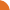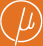Chair for Foundations of Software Reliability and Theoretical Computer Science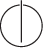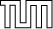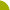Publications - On Finite Monoids over Nonnegative Integer Matrices and Short Killing WordsReference: Stefan Kiefer and Corto Mascle. On finite monoids over nonnegative integer matrices and short killing words. In Proceedings of the 36th International Symposium on Theoretical Aspects of Computer Science (STACS), Leibniz International Proceedings in Informatics (LIPIcs), pages 43:1–43:13, 2019. Abstract: Let n be a natural number and M a set of n x n-matrices over the nonnegative integers such that M generates a finite multiplicative monoid. We show that if the zero matrix 0 is a product of matrices in M, then there are M_1, ..., M_n^5 in M with M_1 *s M_n^5 = 0. This result has applications in automata theory and the theory of codes. Specifically, if X subset Sigma^* is a finite incomplete code, then there exists a word w in Sigma^* of length polynomial in sum_x in X |x| such that w is not a factor of any word in X^*. This proves a weak version of Restivo's conjecture. Suggested BibTeX entry: @inproceedings{19KM-STACS,     author = {Stefan Kiefer and Corto Mascle},     booktitle = {Proceedings of the 36th International Symposium on Theoretical Aspects of Computer Science (STACS)},     pages = {43:1--43:13},     series = {Leibniz International Proceedings in Informatics (LIPIcs)},     title = {On Finite Monoids over Nonnegative Integer Matrices and Short Killing Words},     year = {2019} }